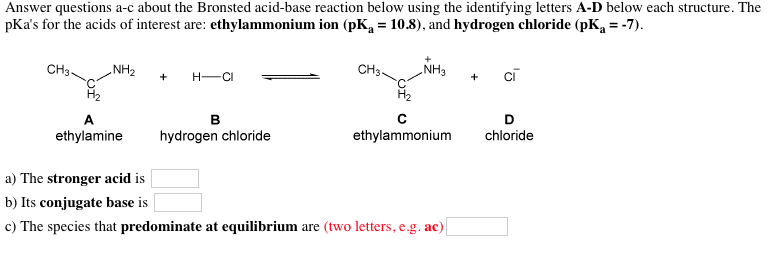# Question & Answer: Answer questions a-c about the Bronsted acid-base reaction below using the identifying letters…..Answer questions a-c about the Bronsted acid-base reaction below using the identifying letters A-D below each structure. The pKa’s for the acids of interest are: ethylammonium ion (pK_a = 10.8), and hydrogen chloride (pK_a = 7) a) The stronger acid is b) Its conjugate base is c) The species that predominate at equilibrium are (two letters, e.g. ac)

CH3CH2NH2 + HCl— > CH3CH2NH3+ + Cl

Don't use plagiarized sources. Get Your Custom Essay on
GET AN ESSAY WRITTEN FOR YOU FROM AS LOW AS \$13/PAGE

A Bronsted-Lowry acid is any species that is capable of donating a proton and a Bronsted-Lowry base is any species that is capable of accepting a proton.

Here, hydrochloric acid (B) donates H+ to ethylamine (A) which accepts it, forming a positively charged ethyl ammonium ion (C) and a negatively charged chloride ion (D). Therefore, HCl is a Bronsted-Lowry acid and ethylamine is a Bronsted-Lowry base. Cl is the conjugate base of the acid HCl and ethyl ammonium ion is the conjugate acid of the base ethylamine

In reaction, there are two Bronsted-Lowry acids, one is HCl and other is ethyl ammonium ion. The PKa of ethyl ammonium ion is 10.8 and PKa of HCl is – 7

Calculate Ka of ethyl ammonium ion

PKa = -log (Ka)

Ka = 10^(-10.8) = 1.58 x 10^-11

Calculate Ka of HCl

PKa = -log (Ka)

Ka = 10^(7) = 1.0 x 10^7

Acid dissociation constant of HCl is greater than acid dissociation constant of ethyl ammonium ion, so HCl is stronger acid.

a) The stronger acid is HCl

b) Its conjugate base. Cl

c) The species that predominant at equilibrium is ec (ethylammonium chloride)